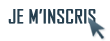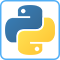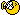IdentifiantMot de passe
Mot de passe oublié ?

Inscrivez-vous gratuitement
pour pouvoir participer, suivre les réponses en temps réel, voter pour les messages, poser vos propres questions et recevoir la newsletterDiscussion :

# Need help can you make my homework ? Python Pandas DataFrame

Sujet :

## Python

1.Need help can you make my homework ? Python Pandas DataFrame
Hey I need help for my homework can someone make my homework for free for me please its very important. The deadline is for 12 september I need have the code and analyse in a jupyter notebook and if its possible get bloc note with your analyse your thinks because I have presentation after.

1. Data cleaning: replace certain identical occurrences, duplicates, etc.

2. Calculate the average price per vehicle to be stored in a database

3. Calculate a price order for the company: cheap, medium, high

4. Perform a simple OLS regression between the target price and the explanatory variable curbweight
Perform statistical interference on the regression coefficient (interpret the results) and verify the structure of the residuals

5. Perform a multiple OLS regression between the target price and 2 explanatory variables curbweight and enginesize on all the data. Perform statistical interference on the regression coefficient and verify the structure of the residuals

6. Encoder all categorical variables

7. Cross-validate using a regression model (take 30% as the size of test_data and verify that the distribution is constant). Calculate the scores on the two samples (train and test). Save the model.

and this csv file: https://file.io/cXT9UgDWjhVy
Thank you !!Répondre avec citation   0  1

2.Hi.

If I understand correctly, you want us to do the code for you?

Here we are developers helping others. We don't code for them.Répondre avec citation   1  0

3.Faut pas pousser mémé avant d'avoir tué le bébé avec l'eau du bain !J'aime bien programmer mais y a des limites !Répondre avec citation   0  0

 Actualités F.A.Q PYTHON TUTORIELS PYTHON SOURCES PYTHON OUTILS PYTHON LIVRES PYTHON PyQt